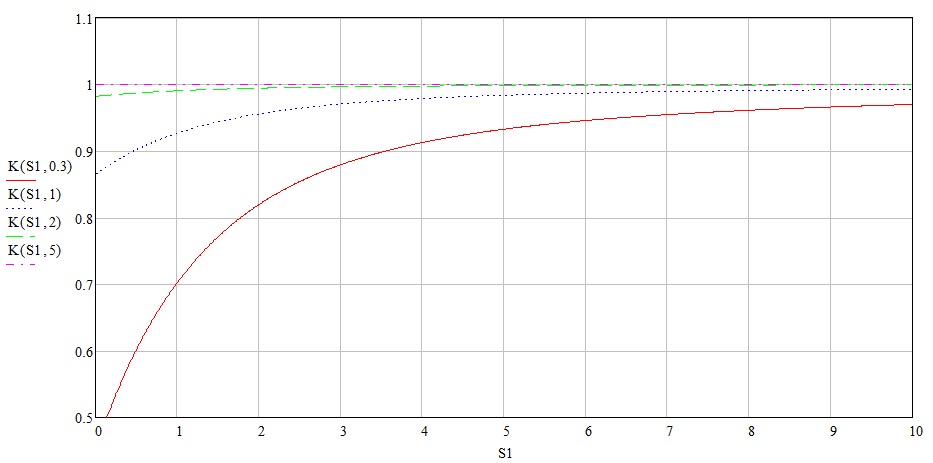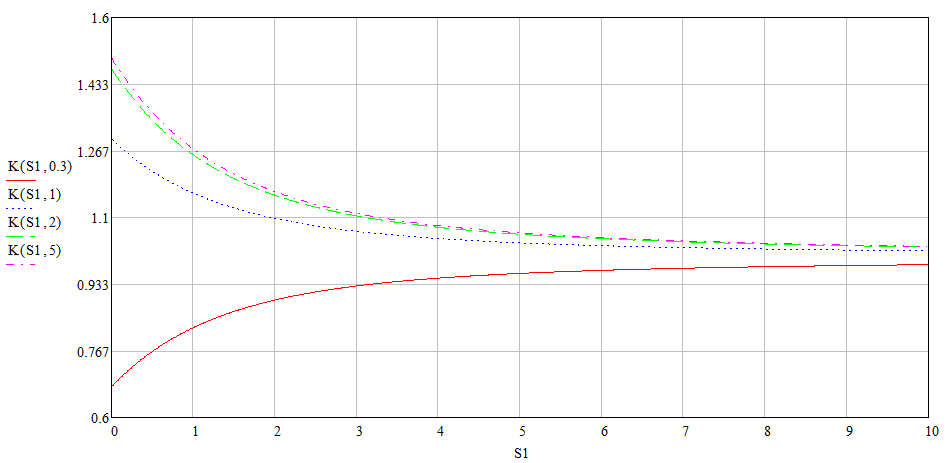Research website of Vyacheslav Gorchilin
2019-08-20
A method of increasing the efficiency of the 2nd kind on the parametric LR-circuit
From the definition of the efficiency of the second kind , it follows that for no cost increase necessary to raise the voltage with the same charge. Known reactive elements this gives inductance, but not directly, but in certain manipulations with this parameter: its parametric changes. If you imagine a coil, which at the time of its pump current, and hence charge, the inductance is one value, and disabling of the pump and the appearance of the back EMF — more and more, we just get a higher voltage with the same charge. To further enhance the effectiveness of this principle it is possible, if you change the resistance in LR-circuit simultaneously with the change in inductance. In itself, the change of this parameter does not lead to increased efficiency.
In this article we will talk about the method, which involved parametric inductance and active resistance in the sequential LR-circuit and the parameter is the time. Here we will focus only on one version, which seems to us the most simple from the point of view of mathematical apparatus and from the standpoint of its implementation, but the same principle it is possible to disperse to other solutions. First, we will examine his math and show the theoretical possibility of increasing $$\eta_{2}$$, and then put it on the actual circuitry of the device.
From formula (4.8) in this work we determined the energy ratio in the parametric circuit. In this case, we specify it to the figure (1a): $W_R + W_F = W_E \qquad (1.1)$ Here: $$W_R$$ — energy dissipated in the active resistance $$R$$, $$W_F$$ is the energy stored or given inductance $$L$$ and $$W_E$$ is the energy from the power source $$U$$. Then the formula that will give us a picture of the increase or decrease in efficiency will obviously be: $K_{\eta 2} = {W_R \over W_E} \qquad (1.2)$ In the case where the inductance does not change, and at first stores energy and then releases energy, the efficiency is equal to one and $$K_{\eta 2} = 1$$. But we are interested in the variant in which $$K_{\eta 2} \gt 1$$, which we will discuss next. First, we recall how to calculate current and energy in the RL-circuits with constant parameters, and then begin to change them in time.Fig.1. The equivalent circuit for the calculations (a,b) and a graph of the current in the inductor (c)
From the theory of transient processes  we know that in a series circuit (Fig. 1a) consisting of a voltage source $$U$$, the inductance $$L$$ and resistance $$R$$, after a circuit of key SW1, the current will be so: $I(t) = {U \over R} (1 - e^{-t/\tau}), \quad \tau = {L \over R} \qquad (1.3)$ Then the energy dissipated in the resistance for the time $$T$$ will be so $W_R = R \int \limits_0^T I(t)^2 \Bbb{d}t \qquad (1.4)$ and the energy consumed from the power source at the same time, so: $W_E = \int \limits_0^T U I(t) \Bbb{d}t \qquad (1.5)$ But if we substitute (1.4-1.5) in (1.2), and everything printeriem, we get $$K_{\eta 2} \le 1$$. But initially we had planned a very different result.
Increase efficiency
Let's try to change the inductance and the resistance in time to achieve the desired result. For this, we calculate the diagram shown in figure (1b) in which two keys SW1 and SW2, operate alternately: the key SW1 connects the chain $$L_1 R_1$$ to the power source in the time interval ($$0, T_1$$), then opens its contacts, and the key SW2 closes and it closes the second circuit $$L_2 R_2$$ in the interval ($$T_1, T$$) where $$T$$ is the time period after which all repeats. Two inductors linked by a single magnetic flux, and because they work in different time periods, we can assume that it is one and the same inductance, changing the parameter in time. Then we have to consider the energy of two processes for the two time intervals (Fig. 1c).
1 interval: $$0 .. T_1$$.
Current for this interval we find from the formula (1.3), which immediately substitute in (1.4-1.5): $W_{R1} = {U^2 \over R_1} \int \limits_0^{T_1} (1 - e^{-t/\tau 1})^2 \Bbb{d}t \qquad (1.6)$ $W_{E1} = U \int \limits_0^T (1 - e^{-t/\tau 1}) \Bbb{d}t, \quad \tau_1 = {L_1 \over R_1} \qquad (1.7)$
2 interval: $$T_1 .. T$$.
At the beginning of the second interval the current in the circuit is maximum of $$I_1$$, and because the power source is switched off here, the decay of this current will be according to another equation: $I(t) = I_1 e^{-t/\tau2}, \quad \tau_2 = {L_2 \over R_2} \qquad (1.8)$ the current will be from (1.3) based on the data of the first interval: $I_1 = {U \over R_1} (1 - e^{-T_1/\tau1}) \qquad (1.9)$ Then the energy circuit for the second interval will look like this: $W_{R2} = I_1 R_2^2 \int \limits_{0}^{T-T_1} e^{-2t/\tau 2} \Bbb{d}t \qquad (1.10)$ $W_{E2} = 0 \qquad (1.11)$ Here we are, as if it is independent of the initial interval with a starting current $$I_1$$, and therefore the limits of integration are taken: ($$0, T-T_1$$). Energy from the power source in this interval is not potreblenia and therefore is equal to zero.
We come to the most interesting — the overall efficiency and the possibility of its increase. From (1.2) that increase/decrease efficiency we can find by dividing the energy dissipated in the resistance for the energy consumed from the power source. In our case, we need to calculate all of this for two intervals $K_{\eta 2} = {W_{R1} + W_{R2} \over W_{E1} + W_{E2}} \qquad (1.12)$ the values of which are respectively from the formulas (1.6-1.7) and (1.10-1.11), after their integration. We will not torture our readers with the calculations and just give the final result: $K_{\eta 2} = {S_1 - 1.5 + 2 e^{-S_1} (1 - 0.25 e^{-S_1}) + 0.5 \delta \left(1 - e^{-S_1}\right)^2 (1 - e^{-2 S_2}) \over S_1 - 1 + e^{-S_1}} \qquad (1.13)$ where: $S_1 = {T_1 \over \tau_1}, \quad S_2 = {T - T_1 \over \tau_2}, \quad \delta = {L_2 \over L_1}$ Needless to say, the entire period must be greater than its parts, i.e.: $$T \gt T_1$$. It can be shown that if the ratio of the inductances will be equal to one (i.e., in both intervals the same inductance), the increase in efficiency will be. Or more generally: $K_{\eta 2} \le 1 \quad \Bbb{if} \quad \delta \le 1 \qquad (1.14)$ But if the inductance of the second interval will be more inductance in the first, then, subject to other parameters, the increase vplone possible.Fig.2. The graph of the variation of the efficiency index from the parameters of the scheme when $$\delta = 1$$Fig.3. The graph of the variation of the efficiency index from the parameters of the scheme when $$\delta = 1.5$$
The graphs in figures (2,3) was built according to the formula (1.13) with the following relationship: $$K = K_{\eta 2}(S_1, S_2)$$. The first one is the efficiency for the circuit without changing the inductance, and the second is an example of the possible efficiency of the second kind with respect to the inductances equal to one and a half. At this stage it becomes obvious that to increase the efficiency of just in need of a change of inductance in a big way when breakage of the pump current, and also, the largest possible value of $$S_2$$, for example, when the second interval is much larger (large duty cycle pulse).
Of course, this calculation is only theoretical value, because it does not account for additional real factors: the resistance of the wires of the coils, feed-through and self-capacitance of the coil, the efficiency of the generator $$U$$, transients of the switching keys. That, and the more realistic circuitry, we will discuss in the next part of this work.

The materials used
1. Transients in RLC-circuits of the first order.